# Lorene::Eos_consistent Class Reference

Equation of state for the CompOSE database with a consistent computation of the log-enthalpy (derived from Eos_CompOSE ). More...

#include <eos_compose.h>

Inheritance diagram for Lorene::Eos_consistent: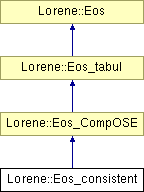List of all members.

## Public Member Functions

Eos_consistent (const string &files_path)
Constructor from CompOSE data.
Eos_consistent (const char *file_name)
Standard constructor.
virtual ~Eos_consistent ()
Destructor.
virtual bool operator== (const Eos &) const
Comparison operator (egality).
virtual bool operator!= (const Eos &) const
Comparison operator (difference).
virtual int identify () const
Returns a number to identify the sub-classe of Eos the object belongs to.
virtual double nbar_ent_p (double ent, const Param *par=0x0) const
Computes the baryon density from the log-enthalpy.
virtual double ener_ent_p (double ent, const Param *par=0x0) const
Computes the total energy density from the log-enthalpy.
virtual double press_ent_p (double ent, const Param *par=0x0) const
Computes the pressure from the log-enthalpy.
virtual bool operator== (const Eos &) const =0
Comparison operator (egality).
virtual bool operator!= (const Eos &) const =0
Comparison operator (difference).
virtual void sauve (FILE *) const
Save in a file.
virtual double der_nbar_ent_p (double ent, const Param *par=0x0) const
Computes the logarithmic derivative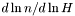from the log-enthalpy.
virtual double der_ener_ent_p (double ent, const Param *par=0x0) const
Computes the logarithmic derivative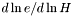from the log-enthalpy.
virtual double der_press_ent_p (double ent, const Param *par=0x0) const
Computes the logarithmic derivative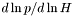from the log-enthalpy.
virtual double der_press_nbar_p (double ent, const Param *par=0x0) const
Computes the logarithmic derivative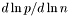from the log-enthalpy.
const char * get_name () const
Returns the EOS name.
void set_name (const char *name_i)
Sets the EOS name.
Cmp nbar_ent (const Cmp &ent, int nzet, int l_min=0, Param *par=0x0) const
Computes the baryon density field from the log-enthalpy field and extra parameters.
Scalar nbar_ent (const Scalar &ent, int nzet, int l_min=0, Param *par=0x0) const
Computes the baryon density field from the log-enthalpy field and extra parameters.
Cmp ener_ent (const Cmp &ent, int nzet, int l_min=0, Param *par=0x0) const
Computes the total energy density from the log-enthalpy and extra parameters.
Scalar ener_ent (const Scalar &ent, int nzet, int l_min=0, Param *par=0x0) const
Computes the total energy density from the log-enthalpy and extra parameters.
Cmp press_ent (const Cmp &ent, int nzet, int l_min=0, Param *par=0x0) const
Computes the pressure from the log-enthalpy and extra parameters.
Scalar press_ent (const Scalar &ent, int nzet, int l_min=0, Param *par=0x0) const
Computes the pressure from the log-enthalpy and extra parameters.
Cmp der_nbar_ent (const Cmp &ent, int nzet, int l_min=0, Param *par=0x0) const
Computes the logarithmic derivativefrom the log-enthalpy and extra parameters.
Scalar der_nbar_ent (const Scalar &ent, int nzet, int l_min=0, Param *par=0x0) const
Computes the logarithmic derivativefrom the log-enthalpy and extra parameters.
Cmp der_ener_ent (const Cmp &ent, int nzet, int l_min=0, Param *par=0x0) const
Computes the logarithmic derivativefrom the log-enthalpy and extra parameters.
Scalar der_ener_ent (const Scalar &ent, int nzet, int l_min=0, Param *par=0x0) const
Computes the logarithmic derivativefrom the log-enthalpy and extra parameters.
Cmp der_press_ent (const Cmp &ent, int nzet, int l_min=0, Param *par=0x0) const
Computes the logarithmic derivativefrom the log-enthalpy and extra parameters.
Scalar der_press_ent (const Scalar &ent, int nzet, int l_min=0, Param *par=0x0) const
Computes the logarithmic derivativefrom the log-enthalpy and extra parameters.

## Static Public Member Functions

static Eoseos_from_file (FILE *)
Construction of an EOS from a binary file.
static Eoseos_from_file (ifstream &)
Construction of an EOS from a formatted file.

## Protected Member Functions

Eos_consistent (FILE *)
Constructor from a binary file (created by the function sauve(FILE*) ).
Eos_consistent (ifstream &)
Constructor from a formatted file.
Reads the file containing the table and initializes in the arrays logh , logp and dlpsdlh .
Reads the files containing the table and initializes in the arrays logh , logp and dlpsdlh (CompOSE format).
virtual ostream & operator>> (ostream &) const
Operator >>.
void calcule (const Cmp &thermo, int nzet, int l_min, double(Eos::*fait)(double, const Param *) const, Param *par, Cmp &resu) const
General computational method for Cmp 's.
void calcule (const Scalar &thermo, int nzet, int l_min, double(Eos::*fait)(double, const Param *) const, Param *par, Scalar &resu) const
General computational method for Scalar 's.

## Protected Attributes

int format
0 for standard (old) LORENE format, 1 for CompOSE format
string tablename
Name of the file containing the tabulated data.
string authors
Authors - reference for the table.
double hmin
Lower boundary of the enthalpy interval.
double hmax
Upper boundary of the enthalpy interval.
Tbllogh
Table of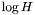.
Tbllogp
Table of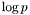.
Tbldlpsdlh
Table of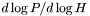.
Tbllognb
Table of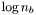.
Tbldlpsdlnb
Table of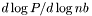.
double * press
double * nb
double * ro
char name 
EOS name.

## Private Member Functions

Eos_consistent (const Eos_consistent &)
Copy constructor (private to make Eos_consistent a non-copiable class).

## Friends

EosEos::eos_from_file (FILE *)
The construction functions from a file.
EosEos::eos_from_file (ifstream &)
ostream & operator<< (ostream &, const Eos &)
Display.

## Detailed Description

Equation of state for the CompOSE database with a consistent computation of the log-enthalpy (derived from Eos_CompOSE ).

General tabulated EOS, reading a table passed as an argument to the constructor. The log-enthalpy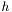is computed to ensure the relation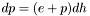, thus eventually modifying the table. When built with Eos::eos_from_file(), the file must be composed of the following lines:

20	Type of the EOS
1	0: standard format	1: CompOSE format
Tabulated EoS
/full/path/to/the/eos/table/name_of_the_table 

On the second line '0' means that the table has the standard LORENE format for tabulated EoSs. '1' means that the files from the CompOSE database are used and that the 'name_of_the_table' should be without suffix: e.g. great_eos would stand for files great_eos.nb and great_eos.thermo (see CompOSE documentation).

Definition at line 216 of file eos_compose.h.

## Constructor & Destructor Documentation

 Lorene::Eos_consistent::Eos_consistent ( const string & files_path )

Constructor from CompOSE data.

Parameters:
 files_path Absolute name (including path), but without extensions of the CompOSE data, e.g. /home/foo/eos/my_eos standing for files my_eos.nb and my_eos.thermo as dowloaded from the CompOSE server.

Definition at line 97 of file eos_consistent.C.

 Lorene::Eos_consistent::Eos_consistent ( const char * file_name )

Standard constructor.

Parameters:
 file_name Absolute name (including path) containing the EOS file. This file should contain a header with the following first lines: # Comments ... # Name of the authors / reference # Comments ... # Comments ... # Comments XXX <-- Number of lines # # index n_B [fm^{-3}] rho [g/cm^3] p [dyn/cm^2] #  where 'XXX' is the number of following lines of the EoS, each line containing an index (integer), baryon density, energy total density and pressure in the units given above.

Definition at line 76 of file eos_consistent.C.

 Lorene::Eos_consistent::Eos_consistent ( FILE * fich )  [protected]

Constructor from a binary file (created by the function sauve(FILE*) ).

This constructor is protected because any EOS construction from a binary file must be done via the function Eos::eos_from_file(FILE*) .

Definition at line 82 of file eos_consistent.C.

 Lorene::Eos_consistent::Eos_consistent ( ifstream & fich )  [protected]

Constructor from a formatted file.

This constructor is protected because any EOS construction from a formatted file must be done via the function Eos::eos_from_file(ifstream& ) .

Definition at line 90 of file eos_consistent.C.

 Lorene::Eos_consistent::Eos_consistent ( const Eos_consistent & )  [private]

Copy constructor (private to make Eos_consistent a non-copiable class).

 Lorene::Eos_consistent::~Eos_consistent ( )  [virtual]

Destructor.

Definition at line 274 of file eos_consistent.C.

## Member Function Documentation

 void Lorene::Eos::calcule ( const Scalar & thermo, int nzet, int l_min, double(Eos::*)(double, const Param *) const fait, Param * par, Scalar & resu ) const [protected, inherited]

General computational method for Scalar 's.

Parameters:
 thermo [input] thermodynamical quantity (for instance the enthalpy field)from which the thermodynamical quantity resu is to be computed. nzet [input] number of domains where resu is to be computed. l_min [input] index of the innermost domain is which resu is to be computed [default value: 0]; resu is computed only in domains whose indices are in [l_min,l_min+nzet-1] . In the other domains, it is set to zero. fait [input] pointer on the member function of class Eos which performs the pointwise calculation. par possible extra parameters of the EOS resu [output] result of the computation.

Definition at line 282 of file eos.C.

 void Lorene::Eos::calcule ( const Cmp & thermo, int nzet, int l_min, double(Eos::*)(double, const Param *) const fait, Param * par, Cmp & resu ) const [protected, inherited]

General computational method for Cmp 's.

Parameters:
 thermo [input] thermodynamical quantity (for instance the enthalpy field)from which the thermodynamical quantity resu is to be computed. nzet [input] number of domains where resu is to be computed. l_min [input] index of the innermost domain is which resu is to be computed [default value: 0]; resu is computed only in domains whose indices are in [l_min,l_min+nzet-1] . In the other domains, it is set to zero. fait [input] pointer on the member function of class Eos which performs the pointwise calculation. par possible extra parameters of the EOS resu [output] result of the computation.

Definition at line 210 of file eos.C.

 Scalar Lorene::Eos::der_ener_ent ( const Scalar & ent, int nzet, int l_min = 0, Param * par = 0x0 ) const [inherited]

Computes the logarithmic derivativefrom the log-enthalpy and extra parameters.

Parameters:
 ent [input, unit: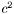] log-enthalpy H defined by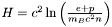, where e is the (total) energy density, p the pressure, n the baryon density, and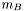the baryon mass nzet number of domains where the derivative dln(e)/dln(H) is to be computed. l_min index of the innermost domain is which the coefficient dln(n)/dln(H) is to be computed [default value: 0]; the derivative dln(e)/dln(H) is computed only in domains whose indices are in [l_min,l_min+nzet-1] . In the other domains, it is set to zero. par possible extra parameters of the EOS
Returns:
dln(e)/dln(H)

Definition at line 461 of file eos.C.

 Cmp Lorene::Eos::der_ener_ent ( const Cmp & ent, int nzet, int l_min = 0, Param * par = 0x0 ) const [inherited]

Computes the logarithmic derivativefrom the log-enthalpy and extra parameters.

Parameters:
 ent [input, unit:] log-enthalpy H defined by, where e is the (total) energy density, p the pressure, n the baryon density, andthe baryon mass nzet number of domains where the derivative dln(e)/dln(H) is to be computed. l_min index of the innermost domain is which the coefficient dln(n)/dln(H) is to be computed [default value: 0]; the derivative dln(e)/dln(H) is computed only in domains whose indices are in [l_min,l_min+nzet-1] . In the other domains, it is set to zero. par possible extra parameters of the EOS
Returns:
dln(e)/dln(H)

Definition at line 451 of file eos.C.

 double Lorene::Eos_tabul::der_ener_ent_p ( double ent, const Param * par = 0x0 ) const [virtual, inherited]

Computes the logarithmic derivativefrom the log-enthalpy.

Parameters:
 ent [input, unit:] log-enthalpy H
Returns:
dln(e)/dln(H)

Implements Lorene::Eos.

Definition at line 485 of file eos_tabul.C.

 Scalar Lorene::Eos::der_nbar_ent ( const Scalar & ent, int nzet, int l_min = 0, Param * par = 0x0 ) const [inherited]

Computes the logarithmic derivativefrom the log-enthalpy and extra parameters.

Parameters:
 ent [input, unit:] log-enthalpy H defined by, where e is the (total) energy density, p the pressure, n the baryon density, andthe baryon mass nzet number of domains where the derivative dln(n)/dln(H) is to be computed. l_min index of the innermost domain is which the coefficient dln(n)/dln(H) is to be computed [default value: 0]; the derivative dln(n)/dln(H) is computed only in domains whose indices are in [l_min,l_min+nzet-1] . In the other domains, it is set to zero. par possible extra parameters of the EOS
Returns:
dln(n)/dln(H)

Definition at line 438 of file eos.C.

 Cmp Lorene::Eos::der_nbar_ent ( const Cmp & ent, int nzet, int l_min = 0, Param * par = 0x0 ) const [inherited]

Computes the logarithmic derivativefrom the log-enthalpy and extra parameters.

Parameters:
 ent [input, unit:] log-enthalpy H defined by, where e is the (total) energy density, p the pressure, n the baryon density, andthe baryon mass nzet number of domains where the derivative dln(n)/dln(H) is to be computed. l_min index of the innermost domain is which the coefficient dln(n)/dln(H) is to be computed [default value: 0]; the derivative dln(n)/dln(H) is computed only in domains whose indices are in [l_min,l_min+nzet-1] . In the other domains, it is set to zero. par possible extra parameters of the EOS
Returns:
dln(n)/dln(H)

Definition at line 428 of file eos.C.

 double Lorene::Eos_tabul::der_nbar_ent_p ( double ent, const Param * par = 0x0 ) const [virtual, inherited]

Computes the logarithmic derivativefrom the log-enthalpy.

Parameters:
 ent [input, unit:] log-enthalpy H
Returns:
dln(n)/dln(H)

Implements Lorene::Eos.

Definition at line 462 of file eos_tabul.C.

 Scalar Lorene::Eos::der_press_ent ( const Scalar & ent, int nzet, int l_min = 0, Param * par = 0x0 ) const [inherited]

Computes the logarithmic derivativefrom the log-enthalpy and extra parameters.

Parameters:
 ent [input, unit:] log-enthalpy H defined by, where e is the (total) energy density, p the pressure, n the baryon density, andthe baryon mass nzet number of domains where the derivative dln(p)/dln(H) is to be computed. par possible extra parameters of the EOS l_min index of the innermost domain is which the coefficient dln(n)/dln(H) is to be computed [default value: 0]; the derivative dln(p)/dln(H) is computed only in domains whose indices are in [l_min,l_min+nzet-1] . In the other domains, it is set to zero.
Returns:
dln(p)/dln(H)

Definition at line 483 of file eos.C.

 Cmp Lorene::Eos::der_press_ent ( const Cmp & ent, int nzet, int l_min = 0, Param * par = 0x0 ) const [inherited]

Computes the logarithmic derivativefrom the log-enthalpy and extra parameters.

Parameters:
 ent [input, unit:] log-enthalpy H defined by, where e is the (total) energy density, p the pressure, n the baryon density, andthe baryon mass nzet number of domains where the derivative dln(p)/dln(H) is to be computed. par possible extra parameters of the EOS l_min index of the innermost domain is which the coefficient dln(n)/dln(H) is to be computed [default value: 0]; the derivative dln(p)/dln(H) is computed only in domains whose indices are in [l_min,l_min+nzet-1] . In the other domains, it is set to zero.
Returns:
dln(p)/dln(H)

Definition at line 473 of file eos.C.

 double Lorene::Eos_tabul::der_press_ent_p ( double ent, const Param * par = 0x0 ) const [virtual, inherited]

Computes the logarithmic derivativefrom the log-enthalpy.

Parameters:
 ent [input, unit:] log-enthalpy H
Returns:
dln(p)/dln(H)

Implements Lorene::Eos.

Definition at line 505 of file eos_tabul.C.

 double Lorene::Eos_tabul::der_press_nbar_p ( double ent, const Param * par = 0x0 ) const [virtual, inherited]

Computes the logarithmic derivativefrom the log-enthalpy.

Parameters:
 ent [input, unit:] log-enthalpy H
Returns:
dln(p)/dln(n)

Definition at line 535 of file eos_tabul.C.

 Scalar Lorene::Eos::ener_ent ( const Scalar & ent, int nzet, int l_min = 0, Param * par = 0x0 ) const [inherited]

Computes the total energy density from the log-enthalpy and extra parameters.

Parameters:
 ent [input, unit:] log-enthalpy H defined by, where e is the (total) energy density, p the pressure, n the baryon density, andthe baryon mass nzet number of domains where the energy density is to be computed. l_min index of the innermost domain is which the energy density is to be computed [default value: 0]; the energy density is computed only in domains whose indices are in [l_min,l_min+nzet-1] . In the other domains, it is set to zero. par possible extra parameters of the EOS
Returns:
energy density [unit: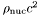], where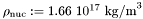Definition at line 394 of file eos.C.

 Cmp Lorene::Eos::ener_ent ( const Cmp & ent, int nzet, int l_min = 0, Param * par = 0x0 ) const [inherited]

Computes the total energy density from the log-enthalpy and extra parameters.

Parameters:
 ent [input, unit:] log-enthalpy H defined by, where e is the (total) energy density, p the pressure, n the baryon density, andthe baryon mass nzet number of domains where the energy density is to be computed. l_min index of the innermost domain is which the energy density is to be computed [default value: 0]; the energy density is computed only in domains whose indices are in [l_min,l_min+nzet-1] . In the other domains, it is set to zero. par possible extra parameters of the EOS
Returns:
energy density [unit:], whereDefinition at line 384 of file eos.C.

References Lorene::Eos::calcule(), Lorene::Eos::ener_ent_p(), and Lorene::Cmp::get_mp().

 double Lorene::Eos_consistent::ener_ent_p ( double ent, const Param * par = 0x0 ) const [virtual]

Computes the total energy density from the log-enthalpy.

Parameters:
 ent [input, unit:] log-enthalpy H
Returns:
energy density e [unit:], whereReimplemented from Lorene::Eos_tabul.

Definition at line 346 of file eos_consistent.C.

 Eos * Lorene::Eos::eos_from_file ( ifstream & fich )  [static, inherited]

Construction of an EOS from a formatted file.

The fist line of the file must start by the EOS number, according to the following conventions:

• 1 = relativistic polytropic EOS (class Eos_poly ).
• 2 = Newtonian polytropic EOS (class Eos_poly_newt ).
• 3 = Relativistic incompressible EOS (class Eos_incomp ).
• 4 = Newtonian incompressible EOS (class Eos_incomp_newt ).
• 5 = Strange matter (MIT Bag model)
• 6 = Strange matter (MIT Bag model) with crust
• 10 = SLy4 (Douchin & Haensel 2001)
• 11 = FPS (Friedman-Pandharipande + Skyrme)
• 12 = BPAL12 (Bombaci et al. 1995)
• 13 = AkmalPR (Akmal, Pandharipande & Ravenhall 1998)
• 14 = BBB2 (Baldo, Bombaci & Burgio 1997)
• 15 = BalbN1H1 (Balberg 2000)
• 16 = GlendNH3 (Glendenning 1985, case 3)
• 17 = Compstar (Tabulated EOS for 2010 CompStar school)
• 18 = magnetized (tabulated) equation of state
• 19 = relativistic ideal Fermi gas at zero temperature (class Eos_Fermi)
• 100 = Multi-domain EOS (class MEos )
• 110 = Multi-polytropic EOS (class Eos_multi_poly )
• 120 = Fitted SLy4 (Shibata 2004)
• 121 = Fitted FPS (Shibata 2004)
• 122 = Fitted AkmalPR (Taniguchi 2005)

The second line in the file should contain a name given by the user to the EOS. The following lines should contain the EOS parameters (one parameter per line), in the same order than in the class declaration.

Definition at line 317 of file eos_from_file.C.

 Eos * Lorene::Eos::eos_from_file ( FILE * fich )  [static, inherited]

Construction of an EOS from a binary file.

The file must have been created by the function sauve(FILE*) .

Definition at line 180 of file eos_from_file.C.

 const char * Lorene::Eos::get_name ( ) const [inherited]

Returns the EOS name.

Definition at line 176 of file eos.C.

References Lorene::Eos::name.

 int Lorene::Eos_consistent::identify ( ) const [virtual]

Returns a number to identify the sub-classe of Eos the object belongs to.

Reimplemented from Lorene::Eos_CompOSE.

Definition at line 164 of file eos_from_file.C.

 Scalar Lorene::Eos::nbar_ent ( const Scalar & ent, int nzet, int l_min = 0, Param * par = 0x0 ) const [inherited]

Computes the baryon density field from the log-enthalpy field and extra parameters.

Parameters:
 ent [input, unit:] log-enthalpy H defined by, where e is the (total) energy density, p the pressure, n the baryon density, andthe baryon mass nzet number of domains where the baryon density is to be computed. l_min index of the innermost domain is which the baryon density is to be computed [default value: 0]; the baryon density is computed only in domains whose indices are in [l_min,l_min+nzet-1] . In the other domains, it is set to zero. par possible extra parameters of the EOS
Returns:
baryon density [unit: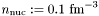]

Definition at line 369 of file eos.C.

 Cmp Lorene::Eos::nbar_ent ( const Cmp & ent, int nzet, int l_min = 0, Param * par = 0x0 ) const [inherited]

Computes the baryon density field from the log-enthalpy field and extra parameters.

Parameters:
 ent [input, unit:] log-enthalpy H defined by, where e is the (total) energy density, p the pressure, n the baryon density, andthe baryon mass nzet number of domains where the baryon density is to be computed. l_min index of the innermost domain is which the baryon density is to be computed [default value: 0]; the baryon density is computed only in domains whose indices are in [l_min,l_min+nzet-1] . In the other domains, it is set to zero. par possible extra parameters of the EOS
Returns:
baryon density [unit:]

Definition at line 359 of file eos.C.

References Lorene::Eos::calcule(), Lorene::Cmp::get_mp(), and Lorene::Eos::nbar_ent_p().

 double Lorene::Eos_consistent::nbar_ent_p ( double ent, const Param * par = 0x0 ) const [virtual]

Computes the baryon density from the log-enthalpy.

Parameters:
 ent [input, unit:] log-enthalpy H
Returns:
baryon density n [unit: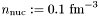]

Reimplemented from Lorene::Eos_tabul.

Definition at line 312 of file eos_consistent.C.

 virtual bool Lorene::Eos::operator!= ( const Eos & ) const [pure virtual, inherited]

Comparison operator (difference).

 bool Lorene::Eos_consistent::operator!= ( const Eos & eos_i ) const [virtual]

Comparison operator (difference).

Reimplemented from Lorene::Eos_CompOSE.

Definition at line 299 of file eos_consistent.C.

References operator==().

 virtual bool Lorene::Eos::operator== ( const Eos & ) const [pure virtual, inherited]

Comparison operator (egality).

 bool Lorene::Eos_consistent::operator== ( const Eos & eos_i ) const [virtual]

Comparison operator (egality).

Reimplemented from Lorene::Eos_CompOSE.

Definition at line 286 of file eos_consistent.C.

References identify(), and Lorene::Eos::identify().

 ostream & Lorene::Eos_consistent::operator>> ( ostream & ost ) const [protected, virtual]

Operator >>.

Reimplemented from Lorene::Eos_CompOSE.

Definition at line 421 of file eos_consistent.C.

 Scalar Lorene::Eos::press_ent ( const Scalar & ent, int nzet, int l_min = 0, Param * par = 0x0 ) const [inherited]

Computes the pressure from the log-enthalpy and extra parameters.

Parameters:
 ent [input, unit:] log-enthalpy H defined by, where e is the (total) energy density, p the pressure, n the baryon density, andthe baryon mass nzet number of domains where the pressure is to be computed. l_min index of the innermost domain is which the pressure is to be computed [default value: 0]; the pressure is computed only in domains whose indices are in [l_min,l_min+nzet-1] . In the other domains, it is set to zero. par possible extra parameters of the EOS
Returns:
pressure [unit:], whereDefinition at line 416 of file eos.C.

 Cmp Lorene::Eos::press_ent ( const Cmp & ent, int nzet, int l_min = 0, Param * par = 0x0 ) const [inherited]

Computes the pressure from the log-enthalpy and extra parameters.

Parameters:
 ent [input, unit:] log-enthalpy H defined by, where e is the (total) energy density, p the pressure, n the baryon density, andthe baryon mass nzet number of domains where the pressure is to be computed. l_min index of the innermost domain is which the pressure is to be computed [default value: 0]; the pressure is computed only in domains whose indices are in [l_min,l_min+nzet-1] . In the other domains, it is set to zero. par possible extra parameters of the EOS
Returns:
pressure [unit:], whereDefinition at line 406 of file eos.C.

 double Lorene::Eos_consistent::press_ent_p ( double ent, const Param * par = 0x0 ) const [virtual]

Computes the pressure from the log-enthalpy.

Parameters:
 ent [input, unit:] log-enthalpy H
Returns:
pressure p [unit:], whereReimplemented from Lorene::Eos_tabul.

Definition at line 386 of file eos_consistent.C.

 void Lorene::Eos_consistent::read_compose_data ( )  [protected, virtual]

Reads the files containing the table and initializes in the arrays logh , logp and dlpsdlh (CompOSE format).

Reimplemented from Lorene::Eos_CompOSE.

Definition at line 186 of file eos_consistent.C.

 void Lorene::Eos_consistent::read_table ( )  [protected, virtual]

Reads the file containing the table and initializes in the arrays logh , logp and dlpsdlh .

Reimplemented from Lorene::Eos_tabul.

Definition at line 102 of file eos_consistent.C.

 void Lorene::Eos_CompOSE::sauve ( FILE * fich ) const [virtual, inherited]

Save in a file.

Reimplemented from Lorene::Eos_tabul.

Definition at line 339 of file eos_compose.C.

References Lorene::Eos_CompOSE::format, and Lorene::fwrite_be().

 void Lorene::Eos::set_name ( const char * name_i )  [inherited]

Sets the EOS name.

Definition at line 170 of file eos.C.

References Lorene::Eos::name.

## Friends And Related Function Documentation

 Eos* Eos::eos_from_file ( FILE * )  [friend]

The construction functions from a file.

Reimplemented from Lorene::Eos_CompOSE.

 ostream& operator<< ( ostream & , const Eos & )  [friend, inherited]

Display.

## Member Data Documentation

 string Lorene::Eos_tabul::authors [protected, inherited]

Authors - reference for the table.

Definition at line 182 of file eos_tabul.h.

 Tbl* Lorene::Eos_tabul::dlpsdlh [protected, inherited]

Table of.

Definition at line 197 of file eos_tabul.h.

 Tbl* Lorene::Eos_tabul::dlpsdlnb [protected, inherited]

Table of.

Definition at line 203 of file eos_tabul.h.

 int Lorene::Eos_CompOSE::format [protected, inherited]

0 for standard (old) LORENE format, 1 for CompOSE format

Definition at line 90 of file eos_compose.h.

 double Lorene::Eos_tabul::hmax [protected, inherited]

Upper boundary of the enthalpy interval.

Definition at line 188 of file eos_tabul.h.

 double Lorene::Eos_tabul::hmin [protected, inherited]

Lower boundary of the enthalpy interval.

Definition at line 185 of file eos_tabul.h.

 Tbl* Lorene::Eos_tabul::logh [protected, inherited]

Table of.

Definition at line 191 of file eos_tabul.h.

 Tbl* Lorene::Eos_tabul::lognb [protected, inherited]

Table of.

Definition at line 200 of file eos_tabul.h.

 Tbl* Lorene::Eos_tabul::logp [protected, inherited]

Table of.

Definition at line 194 of file eos_tabul.h.

 char Lorene::Eos::name [protected, inherited]

EOS name.

Definition at line 199 of file eos.h.

 string Lorene::Eos_tabul::tablename [protected, inherited]

Name of the file containing the tabulated data.

Definition at line 180 of file eos_tabul.h.

The documentation for this class was generated from the following files:

Generated on 7 Dec 2019 for LORENE by1.6.1